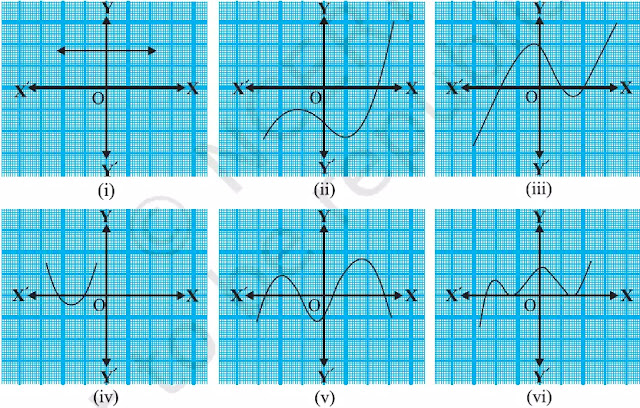# Polynomials

### Important point

For a cubic polynomial,

## Exercise – 2.1

1. The graphs of y=p(x) are given in following figure 2.10, for some polynomials p(x), Find the number of zeroes of p(x), in each case.figure 2.10

solution:

(i) The number of zeroes is 0, because the graph does not intersect the x-axis at any point.

(ii) The number of zeroes is 1, because the graph intersects the x-axis at a point.

(iii) The number of zeroes is 3, because the graph intersects the x-axis at three points.

(iv) The number of zeroes is 2, because the graph intersects the x-axis at two points.

(v) The number of zeroes is 4, because the graph intersects the x-axis at four points.

(vi) The number of zeroes is 3, because the graph intersects the x-axis at three points.